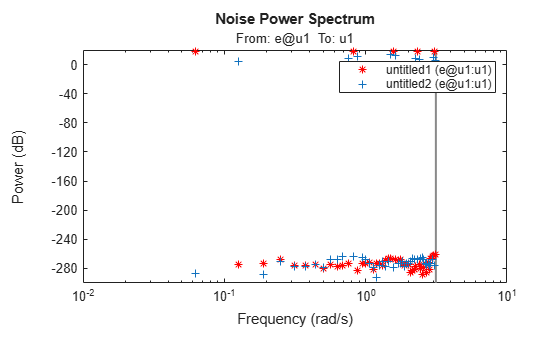spectrum

Plot or return output power spectrum of time series model or disturbance spectrum of linear input-output model

Description

Plot Results

example

spectrum(sys) plots the output power spectrum of an identified time series model sys or the disturbance spectrum of an identified input-output model sys. The function chooses the frequency range and number of points automatically.

• If sys is a time series model, then sys represents the system:

$y\left(t\right)=He\left(t\right)$

Here, e(t) is a Gaussian white noise and y(t) is the observed output.

spectrum plots |H'H|, scaled by the variance of e(t) and the sample time.

• If sys is an input-output model, sys represents the system:

$y\left(t\right)=Gu\left(t\right)+He\left(t\right)$

Here, u(t) is the measured input, e(t) is Gaussian white noise and y(t) is the observed output.

In this case, spectrum plots the spectrum of the disturbance component He(t).

For discrete-time models with sample time Ts, spectrum uses the transformation $z={e}^{j\omega {T}_{s}}$ to map the unit circle to the real frequency axis. The function plots the spectrum only for frequencies smaller than the Nyquist frequency π/Ts, and uses the default value of 1 time unit when Ts is unspecified.

example

spectrum(sys,{wmin, wmax}) creates a spectrum plot for frequencies ranging from wmin to wmax.

spectrum(sys,w) creates a spectrum plot using the frequencies specified in the vector w.

example

spectrum(sys1,...,sysN,w) creates a spectrum plot of several identified models on a single plot. The w argument is optional.

You can specify a color, line style and marker for each model. For example, spectrum(sys1,'r',sys2,'y--',sys3,'gx') uses red for sys1, yellow dash markers for sys2, and green x markers for sys3.

Return Results

ps = spectrum(sys,w) returns the power spectrum amplitude of sys for the specified frequencies, w. There is no plot display.

ps = spectrum(sys,w) returns the power spectrum amplitude of sys for the specified frequencies, w.

[ps,wout] = spectrum(sys) returns the frequency vector, wout, for which the output power spectrum is computed.

[ps,wout,sdps] = spectrum(sys) returns the estimated standard deviations of the power spectrum.

Examples

collapse all

Estimate a fourth-order AR model using a least-squares approach.

sys = ar(z9,4,'ls');

Plot the output spectrum of the model.

spectrum(sys);Estimate a single-input single-output state-space model.

sys = n4sid(z1,2);

Plot the noise spectrum for the model. Specify a frequency range from 0.1 to 50 rad/sec.

spectrum(sys,{0.1,50});The function plots the spectrum, but limits the frequency range to the Nyquist frequency of approximately 31.4 rad/sec.

Create an input consisting of the sum of five sinusoids, each spread over the full frequency range. Compare the spectrum of this signal with that of its square.

Create a sum-of-sinusoids input that extends for 20 periods, with each period containing 100 samples. Specify that the signal combine 5 sinusoids of random phase, using 10 trials to find the set with the lowest signal spread. For more information on this step, see idinput.

u = idinput([100 1 20],'sine',[],[],[5 10 1]);

Create an input-only iddata object u that contains the input u and has a period of 100.

u = iddata([],u,1,'per',100);

Square the input values and store them in new iddata object u2.

u2 = u.u.^2;
u2 = iddata([],u2,1,'per',100);

Use etfe to estimate empirical transfer function models from u and u2. Plot the power spectra of these models together. Use different marker colors and types to distinguish the spectrum sources.

spectrum(etfe(u),'r*',etfe(u2),'+')The plot shows some frequency splitting where the u2-based spectrum does not line up with the u1-based spectrum, but instead, contains two spectral points that flank certain u1-based points. This indicates the nonlinearity of the squared system.

Input Arguments

collapse all

Identified model, specified as an idpoly object, an idproc object, an idss object, or an idtf object.

• If sys is a time series model, then sys represents the system:

$y\left(t\right)=He\left(t\right)$

Here, e(t) is a Gaussian white noise and y(t) is the observed output.

• If sys is an input-output model, then sys represents the system:

$y\left(t\right)=Gu\left(t\right)+He\left(t\right)$

Here, u(t) is the measured input, e(t) is a Gaussian white noise and y(t) is the observed output.

Minimum frequency of the frequency range for which to plot the spectrum, specified as a positive number.

Specify wmin in rad/TimeUnit, where TimeUnit is sys.TimeUnit.

Maximum frequency of the frequency range for which to plot the spectrum, specified as a positive number. By default, the function uses the Nyquist frequency of sys as wmax.

Specify wmax in rad/TimeUnit, where TimeUnit is sys.TimeUnit. If you specify wmax to be greater than the Nyquist frequency, then spectrum will use the Nyquist frequency instead.

Frequencies for which to plot the spectrum, specified as a vector of positive numbers.

Specify w in rad/TimeUnit, where TimeUnit is sys.TimeUnit.

Output Arguments

collapse all

Power spectrum amplitude returned as a numeric vector or a numeric array.

• For single-output models, ps is a 1-by-1- Nw array, where Nw is the length of the frequency vector.

• For multiple-output models, ps is an Ny-byNy-byNw array, where Ny is the number of outputs. ps(:,:,k) corresponds to the power spectrum for the frequency at w(k).

For amplitude values in dB, type psdb = 10*log10(ps).

Frequencies for which the spectrum is plotted, returned as a numeric vector. If you supply w as an input argument, the function returns the identical vector in wout.

Estimated standard deviation of the power spectrum, returned as an array with the same dimensions as ps.

Introduced in R2012a

System Identification Toolbox DocumentationGet trial now